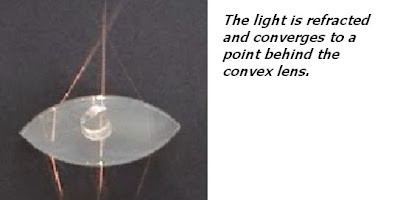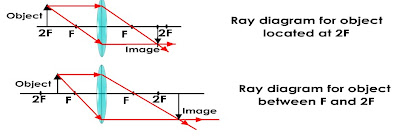## Saturday, September 11, 2010

### reply:How can we remember the characteristics of the convex lenses

dear SBP Student:
Did you mean characteristic of images of convex lens ? If you can't remember is a good idea to draw your ray diagrams to find out the image characteristic at particular situation.

What happens to the light when it passes through the convex lens ?Just follow a few simple steps:-

Step 1:
Draw a ray from the top of the image parallel to the principal axis. This ray bends at the lens axis and goes through the principal focus.Step 2:
Draw a ray from the top of the lens through the centre of the lens.Step 3 :

Where the two rays meet, that is where the image is found.This diagram shows where an object is at a distance between F and 2F. The image is inverted (upside down), real, and diminished (smaller).

Question :
1. What is the image like if the object is at 2F?
2. What is the image like if the object is between F and 2F?
3. What is the image like if the object is at F?
4. What is the image like if the object is less than F?

1 and 23.Image is real, inverted, at infinity.

summary so far## Friday, September 3, 2010

### Selamat Hari Raya AidilFitri

Selamat Hari Raya AidilFitri to all my Muslim friends.